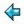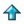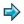### queens.m

```solns = layn(map show (queens 8))
queens 0 = [[]]
queens (n+1) = [q:b|b<-queens n;q<-[1..8];safe q b]
safe q b = and[~checks q b i|i<-index b]
checks q b i = q=b!i \/ abs(q-b!i)=i+1
```

This generates all solutions to the 8 queens problem -- say

```      solns
```
and it will print the solutions one per line - all 92 of them. This is a good program for testing the garbage collector. Say `/gc' to switch on garbage collector diagnostics.

Note that the function `queens n' returns a list of all solutions to the n queens problem (placing queens in the first n columns of a chess board, so that no queen gives check to another). A board with n queens is represented as a list of n numbers, namely the positions of the queens in each column

This example exhibits a basic technique of lazy functional programming, which is to eliminate backtracking from a search problem by working at the level of a list of all solutions, rather than a single solution.